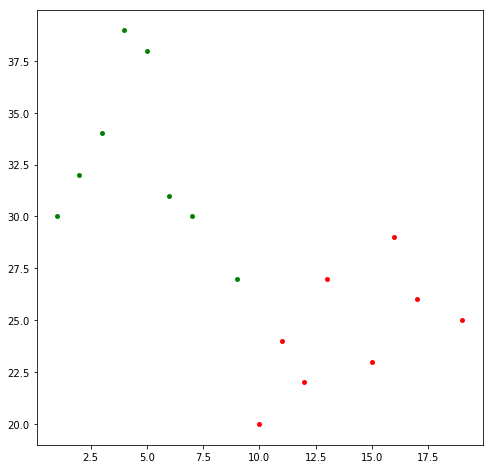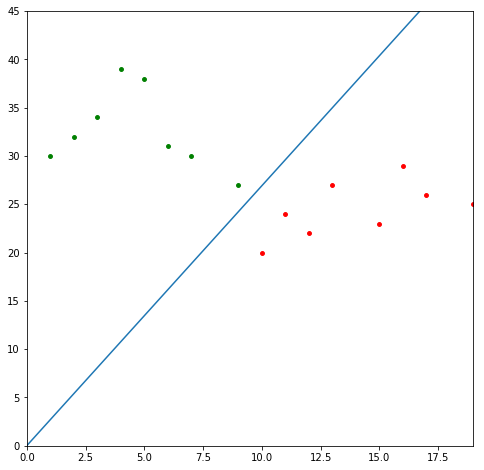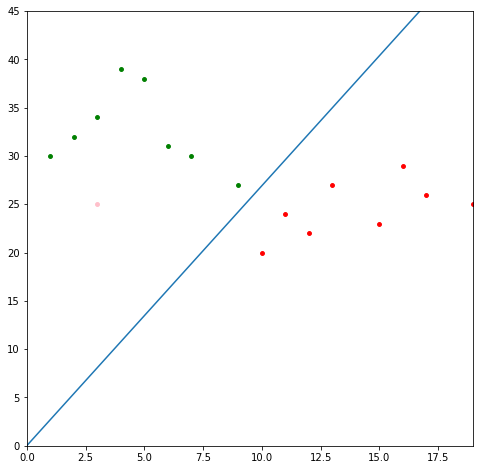# Perceptron Learning Algorithm¶

In the tutorial about SVMs we learned that data can easily be classified with the Perceptron Learning algorithm. The algorithm tries to find a hyperplane/plane/line to separate the data. This is possible if there are two classes and if the data is linearly separable. If this is the case then the data points of one class are on one side of the hyperplane and the data points of the other class are on the other side of the hyperplane. The Perceptron is also the simplest possible Neural Network. Thus, if we understand how this algorithm works, we understand the very basic idea of the mechanism behind SVMs and Neural Networks and we kill two birds with one stone. Hurray! So let's get started and learn how this algorithm can be implemented from scratch. Wait, one more thing before we start: this tutorial, as well as the tutorial about SVMs is mostly inspired by this amazing tutorial: https://www.svm-tutorial.com/ and an amazing book written by the same person (Alexandre Kowalczyk) https://www.svm-tutorial.com/2017/10/support-vector-machines-succinctly-released/
Ok, now we can start for real!

## Data and Data Visualization¶

As always we need some data. Let's create some simple data: data points that belong to the "green" class and data points that belong to the "red" class. Let's also define our target variable: Instances belonging to the green class get the label 1 and instances belonging to the red class get the label -1.

In :
import numpy as np

#Create data for the green class
class_green = np.array([[1, 30], [2,32], [3,34], [5,38], [7,30], [6,31], [9,27],[4,39]])

#Create the target values (1) for the instances of the green class
y_green = np.ones(len(class_green))

#Create data for the red class
class_red = np.array([[10,20], [12,22], [15, 23], [19,25], [13,27], [17,26], [16,29], [11,24]])

#Create the target values (-1) for the instances of the red class
y_red = np.ones(len(class_red))*(-1)


Of course, we would also like to visualize the data. Therefore, let's make a scatter plot! Nice! We can see that a line could easily classify this data.

In :
import matplotlib.pyplot as plt

#definiton of figure size
fig=plt.figure(figsize=(8, 8))

#scatter plot of green data points
plt.scatter(class_green[:,0],class_green[:,1], c="green",  marker='o', s=15)

#scatter plot of red data points
plt.scatter(class_red[:,0],class_red[:,1],  c="red",  marker='o', s=15)

Out:
<matplotlib.collections.PathCollection at 0x115651748>## Hyperplane Equation¶

Great! Finally we have data to work on. So, let's think about our next step - let's find a hyperplane! Well, lucky for us that we don't need to find a hyperplane - a line is enough. In order to find this line let's first recall what the equation for a line looks like:

$y = mx+b$

We just rename y and x as $x_1$ and $x_2$:
$x_2 = mx_1+b$

This is equivalent to:

$0 = mx_1-x_2+b$

Why did we do this? Because we usually have more dimensional data. Thus, we usually work with vectors. For example, if we take an instance of the green class: [2,32] then $x_1 = 2$ and $x_2 = 3$. We can also rewrite this as a vector: $\overrightarrow{x} = (2,32)$. If we define two vectors as $\overrightarrow{x} = (x_1,x_2)$ and $\overrightarrow{w} = (m,-1)$ we get an other line equation where $\overrightarrow{w}\cdot\overrightarrow{x}$ is the dot product of $\overrightarrow{w}$ and $\overrightarrow{x}$:

$\overrightarrow{w}\cdot\overrightarrow{x}+b=0$

Ok, so we got from an equation of a line to a hyperplane equation. Now, let's do it the other way around: Given two vectors $\overrightarrow{w}=(w_0,w_1)$ and $\overrightarrow{x}=(x,y)$ and $b$ we can define a hyperplane:

$\overrightarrow{w}\cdot\overrightarrow{x}+b=0$

This is equivalent to

$w_0x+w_1y+b=0$

when we isolate $y$ we get

$y=-\frac{w_0}{w_1}x-\frac{b}{w_1}$

Then we define $a$ and $c$

$a = -\frac{w_0}{w_1}$ and $c=-\frac{b}{w_1}$

we get

$y=ax+c$

Great! Now we understand the hyperplane equation! We will use the last part of this derivation at the end of the tutorial when we plot the line that separates our data. At least if we find one, of course, but let's stay positive!

As a next step, let's combine our green and red instances as X and their target variable as y.

In :
#combine green and red data points
X = np.vstack((class_green, class_red))

#combine target variables of green and red instances
y= np.hstack((y_green, y_red))


## Hypothesis function¶

Before we define the hypothesis function, let's go back to the hyperplane (in our case the line) equation in order to derive the hypothesis function:
$\overrightarrow{w}\cdot\overrightarrow{x}+b=0$
Imagine we already found the perfect values for $\overrightarrow{w}$ and $b$. If we plugged the values of a new instance $\overrightarrow{x}$ into the equation then the result would probably not be zero but either be a positive value if the instance is above the line (if the instance belongs to the green class) or a negative value if the instance is below the line (if the instance belongs to the red class). If the result was zero then the instance would be on the line.
This is exactly what the hypothesis function does: if the result is positive or zero it gets the label 1 (green class) and if the result is negative it gets the label -1 (red class):

$h(\overrightarrow{x_i}) = \begin{cases}+1 & if & \overrightarrow{w}\cdot \overrightarrow{x} +b \geq 0\\-1 & if & \overrightarrow{w}\cdot \overrightarrow{x} +b < 0\end{cases}$

Nevertheless, this hypothesis function can still be simplified. We don't actually have to write the $+b$ in the end! Do you already have an idea how we can do that? No? No problem! We'll get there:
Given two vectors $\overrightarrow{x} = (x_1,x_2)$ and $\overrightarrow{w} = (w_1,w_2)$ the equation would look like this:

$\overrightarrow{w}\cdot \overrightarrow{x}+b=w_1x_1+w_2x_2+b$

Nevertheless, we could just simply add one value to our vectors instead: $\overrightarrow{x} = (1,x_1,x_2)$ and $\overrightarrow{w} = (b,w1,w2)$ which results in exactly the same equation:

$\overrightarrow{w}\cdot \overrightarrow{x}+b=w_1x_1+w_2x_2+1b$

So, let's do it! Let's add a $1$ to all the feature vectors of our instances:

In :
#add a 1 to each feature vector
X_augmented = np.c_[np.ones(X.shape), X]
print(X_augmented)

[[ 1.  1. 30.]
[ 1.  2. 32.]
[ 1.  3. 34.]
[ 1.  5. 38.]
[ 1.  7. 30.]
[ 1.  6. 31.]
[ 1.  9. 27.]
[ 1.  4. 39.]
[ 1. 10. 20.]
[ 1. 12. 22.]
[ 1. 15. 23.]
[ 1. 19. 25.]
[ 1. 13. 27.]
[ 1. 17. 26.]
[ 1. 16. 29.]
[ 1. 11. 24.]]


Great! Before we start trying to find our vector $\overrightarrow{w}$ we can once more simplify the equation:

$h(\overrightarrow{x_i}) = \begin{cases}+1 & if & \overrightarrow{w}\cdot \overrightarrow{x} \geq 0\\-1 & if & \overrightarrow{w}\cdot \overrightarrow{x} < 0\end{cases}$

After finding the perfect hyperplane, the only thing we need to know is if the result is positive or negative after plugging in the feature values of a new instance into the hyperplane equation. Therefore, the above hyperplane equation is equivalent to:

$h(\overrightarrow{x_i}) = sign(\overrightarrow{w}\cdot \overrightarrow{x})$

Let's have a look at how this looks like in Python!

Easy!

In :
#hypothesis function
def hypothesis(w,x):
return np.sign(np.dot(w,x))


## Finding the Hyperplane¶

As already mentioned, we predict a new instance's class membership by plugging in its values into the hyperplane equation. The only problem is that so far we don't have the values for the hyperplane equation. Therefore, let's find out how to find values for $\overrightarrow{w}$ that classify each of our instances (green or red) correctly. As is so often the case, we start with some random values for $\overrightarrow{w}$ and then try to improve those values step by step by each iteration of the PLA until we find values that define a hyperplane that classifies each instance correctly.
How do we find out whether our values for $\overrightarrow{w}$ were good or bad? Well, we just plug them into the hypothesis equation together with our data and then compare the prediction results with the actual target values of our instances.
We then return all misclassified examples. Let's have a look at how this looks like in Python:

In :
#predict class memebership and return misclassified instances
def predict(hypothesis_function,w,X_augmented,y):
y_pred = np.apply_along_axis(hypothesis_function, 1, X_augmented, w)
misclassified = X_augmented[np.array(y_pred)!=y]
return misclassified


Great! The above function returns all the misclassified instances (in case there are any, which is the case if the values for $\overrightarrow{w}$ were not yet the right ones). Our next step is to randomly pick one of these misclassified instances and return it together with its expected $y$ value (-1 in case the predicted value was 1 and the other way around)

In :
#Randomly pick and return one misclassified instance with its target value from all misclassified instances
def pick_one_from(misclassified_examples, X_augmented, y):
np.random.shuffle(misclassified_examples)
x=misclassified_examples
index = np.where(np.all(X_augmented==x, axis = 1))
return x, y[index]



Ok, so if there are misclassified examples our values for $\overrightarrow{w}$ were apparently not yet the right ones. How do we change them in order to get better values?
First, let's recall from our algebra courses what a dot product actually is:

$\overrightarrow{w}\cdot\overrightarrow{x}=w_1x_1+w_2x_2=||w||||x||cos(\theta)$

where $||w||$ and $||x||$ are the magnitudes of the vectors $\overrightarrow{w}$ and $\overrightarrow{x}$.

Ok, so the dot product tells us something about how two vectors relate and something about the angle between them. How does this help us? Well, apparently the relation and the angle between our vectors were not right in order to make correct predictions. How do we change this?
Ok, I give a small hint: when we subtract two vectors, the angle between them becomes wider. By adding two vectors the angle between them becomes narrower. If the predicted label is 1, then the angle between $\overrightarrow{w}$ is smaller than $90°$ and we want to increase it. If the predicted label is -1, then the angle between $\overrightarrow{w}$ is bigger than $90°$ and we want to decrease it
Thus, if we want to update our values for $\overrightarrow{w}$, we do:

if expected label is +1 we set $\overrightarrow{w}+\overrightarrow{x}$

if expected label is -1 we set $\overrightarrow{w}-\overrightarrow{x}$

We like to simplify everything so instead of making an if else statement we just multiply the sum with the expected value which results in a subtraction in case the expected value is -1:

$\overrightarrow{w}=\overrightarrow{w}+\overrightarrow{x}y_i$

where $y_i$ is the expected value ($1$ or $-1$).

Let's have a look at how this looks like in Python!

Wow, again super easy!

In :
#update and return new values for vector w
def update_rule(expected_y, w, x):
w = w+x*expected_y
return w


Ok, now are finally prepared to write our Perceptron Learning Algorithm:
We initialize $\overrightarrow{w}$ with random values and use these values to make predicitons. Most certainly some of these predcitions or even all of them went wrong. We pick a random misclassfied example and update $\overrightarrow{w}$ with the instance $\overrightarrow{x_i}$. We repeat this procedure until there are no more misclassified examples and return the values for $\overrightarrow{w}$. Let's have a look at how to implement this in Python:

In :
#change values of vector w until every instance is correctly classified then return w
def perceptron_learning_algorithm(X_augmented,y):
w = np.random.rand(3)
misclassified_examples = predict(hypothesis,w,X_augmented,y)
while misclassified_examples.any():
x, expected_y = pick_one_from(misclassified_examples, X_augmented, y)
w = update_rule(expected_y, w, x)
misclassified_examples = predict(hypothesis,w,X_augmented,y)
return w


## The line¶

Ok, now we let our algorithm find values for $\overrightarrow{w}$.

In :
#The inital random values for w stay the same with this random seed
np.random.seed(42)

#get values for w
w = perceptron_learning_algorithm(X_augmented,y)
print(w)

[ -0.62545988 -53.04928569  19.73199394]


Ok, so we found a vector $\overrightarrow{w}$. Now we want to find out if it actually classifies our data correctly. Do you remember when I told you that we would still need the last part of the derivation of how to get to the line equation from the hyperplane equation? Here we go! We arrived at the point where

$y=-\frac{w_0}{w_1}x-\frac{b}{w_1}$

Then we defined $a$ and $c$

$a = -\frac{w_0}{w_1}$ and $c=-\frac{b}{w_1}$

and got

$y=ax+c$

We will use this last part of the derivation now in order to find values for our line:

In :
#calculate value for a
a =-w/w

#calculate value for c
c = -w/w

#create list with numbers ranging from -10 to 50 as x
x = np.array([x+1 for x in range(-10,50)])

#get values for y using the line equation
y=a*x+c


Now, let's plot our line!

Great! It separates our data correctly into green and red instances!

In :
import matplotlib.pyplot as plt

#definiton of figure size
fig=plt.figure(figsize=(8, 8))

#scatter plot of green data points
plt.scatter(class_green[:,0],class_green[:,1], c="green",  marker='o', s=15)

#scatter plot of red data points
plt.scatter(class_red[:,0],class_red[:,1],  c="red",  marker='o', s=15)

#line plot
plt.plot(x, y)

#definition of section to be displayed
plt.axis([0,19,0,45])

Out:
[0, 19, 0, 45]Let's make a test and add another instance to the plot. It has the label 1 and, therefore, the green class membership. Nevertheless, we will give it the colour pink to be able to see which instance we added:

In :
import matplotlib.pyplot as plt

test_instance= np.array([3,25])

#definiton of figure size
fig=plt.figure(figsize=(8, 8))

#scatter plot of green data points
plt.scatter(class_green[:,0],class_green[:,1], c="green",  marker='o', s=15)

#scatter plot of red data points
plt.scatter(class_red[:,0],class_red[:,1],  c="red",  marker='o', s=15)

#scatter plot of new instance
plt.scatter(test_instance, test_instance,  c="pink",  marker='o', s=15)

#line plot
plt.plot(x, y)

#definition of section to be displayed
plt.axis([0,19,0,45])

Out:
[0, 19, 0, 45]Ok, let's see if we predict this correctly with our $\overrightarrow{w}$:
The dot product returns a value above zero which means it gets the label +1. This is also what our hypothesis function predicts. Hurray!

In :
#add 1 as first position of the test isntace's feature vector
test_instance_augmented= np.array([1,3,25])

#Result of dot product of vector w and test instance
y_test = np.dot(w,test_instance_augmented)
print(y_test)

#Prediction of class memebership for test instance
y_test_pred = hypothesis(w, test_instance_augmented)
print(y_test_pred)

333.5265315833624
1.0


Maybe you were a little bit disappointed when you saw the line that classifies our data. It is very close to a green instance. This means that it is likely to misclassify a green instance as red. Actually, there is an infinite number of possible lines that could separate our data and we just found one of them. If we rerun this algorithm and change the random seed that we set to 42 (which means the initial random values for $\overrightarrow{w}$ will become different ones) then this line will look different each time we run this algorithm. Maybe you remember that SVMs solve this problem by finding a hyperplane with the widest margin (the hyperplane with the greatest distance to the nearest data points on both sides). Unfortunately explaining exactly how this is done exceeds the capacity of this tutorial but if you are interested, the book that I recommended at the beginning of this tutorial explains this in a very gentle way. Important to remember is also that this algorithm only works for linearly separable data. Nevertheless, understanding this algorithm helps to understand the very basic idea of what SVMs do and what a perceptron does, the first of all Neural Networks.
I hope you liked this tutorial! There will be more soon!